Courses

# Important definitions and formulas - Statistics Class 10 Notes | EduRev

## Class 10 : Important definitions and formulas - Statistics Class 10 Notes | EduRev

The document Important definitions and formulas - Statistics Class 10 Notes | EduRev is a part of the Class 10 Course Mathematics (Maths) Class 10.
All you need of Class 10 at this link: Class 10

⇒ The three measures of central tendency are:
(a) Mean
(b) Median
(c) Mode
⇒ Mean can be calculated by the following methods:
(a) Direct Method
(b) Short-cut Method
(c) Step Deviation Method
⇒ The mean can be calculated by the following formulae :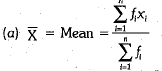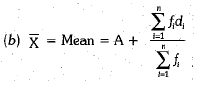where A = assumed mean and di = xi - A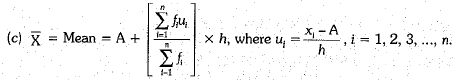⇒ The median of the distribution is the middle value of a distribution.
⇒ The median of a grouped frequency distribution can be calculated by: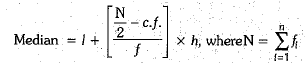l = lower limit of median class
l = frequency of median class
h = width of median class
c.f. = cumulative frequency of the class preceding the median class.
⇒ Mode is the value of the variable which has the maximum frequency.
⇒ The mode of a continuous frequency distribution may be computed by the following formulae:
Mode =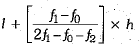where
l = lower limit of the modal class.
f1 = frequency of modal class.
f2 = frequency of class succeeding the modal class.
fo = frequency of class preceding the modal class.
h = size of the class interval.
⇒ The relation between the three measures of central tendency is
Mode = 3 Median - 2 Mean OR 3 Median = Mode + 2 Mean.
⇒ Ogives are used to get the median of a frequency distribution

Offer running on EduRev: Apply code STAYHOME200 to get INR 200 off on our premium plan EduRev Infinity!

## Mathematics (Maths) Class 10

51 videos|346 docs|103 tests

,

,

,

,

,

,

,

,

,

,

,

,

,

,

,

,

,

,

,

,

,

;